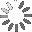# Example sentences for: amortization

How can you use “amortization” in a sentence? Here are some example sentences to help you improve your vocabulary:

• Under this method, the amortization amount of the subsidy cost allowance equals the effective interest minus the nominal interest of the direct loans times the effective interest rate (the discount rate).

• If neither fair value is determinable, the cost of the PP&E acquired is the cost recorded for the PP&E surrendered net of any accumulated depreciation or amortization.

• INTEREST METHOD - (1) Under the interest method of amortization, an amount of interest equal to the carrying amount of the investment times the effective interest rate is calculated for each accounting period.

• AMORTIZATION -The gradual extinguishment of any amount over a period of time through a systematic allocation of the amount over a number of consecutive accounting periods such as the retirement of a debt by serial payments to a sinking fund.

• HISTORICAL COST - Initially, the amount of cash (or its equivalent) paid to acquire an asset; subsequent to acquisition, the historical amount may be adjusted for amortization.

## Search

Search for example sentencesLoading...